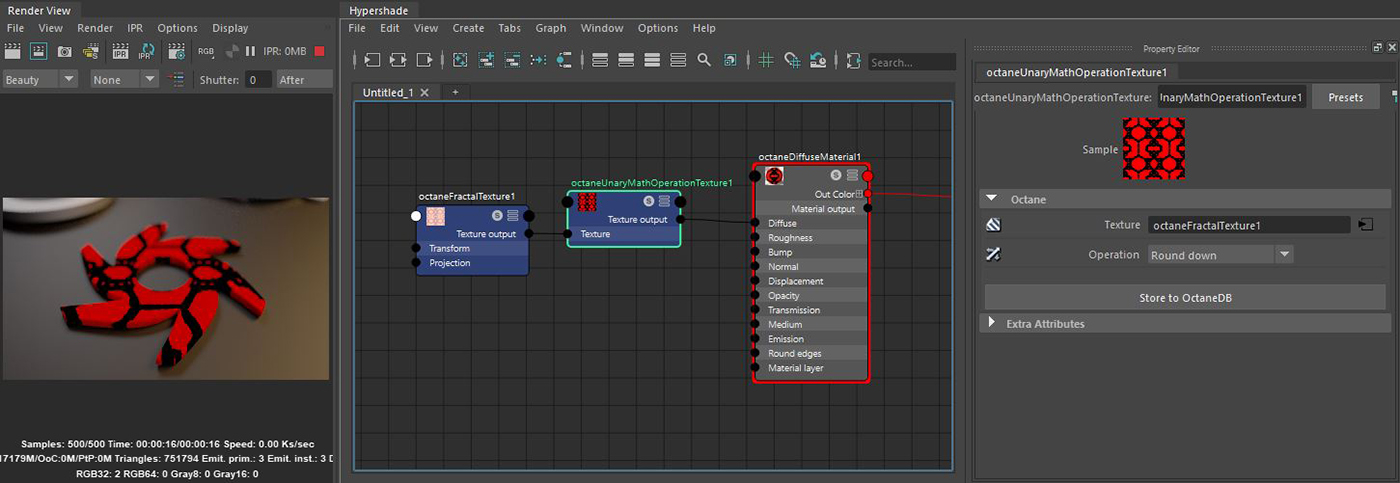# Unary Math Operation Texture

The Unary Math node takes a single input and performs a mathematical calculation on the input determined in the Operation parameter (figure 1).Figure 1: A Fractal Noise texture's contrast has been increased using the Round Down operation available with the Unary Math Operation node

### Unary Math Operation Texture Attributes

Texture - The input argument for the operation.

Operation - The calculation to be performed on the arguments.